Math Courses / Course / Chapter

# Triangle Congruence in Geometry: ASA, SAS, and SSS Postulates.

• Author

Joao Amadeu has more than 10 years of experience in teaching physics and mathematics at different educational levels. Joao earned two degrees at Londrina State University: B.S. in Physics and M.S. in Science and Mathematics Education. He is currently working on his PhD in Science Education at Western Michigan University.

• Instructor
Jeff Calareso

Jeff teaches high school English, math and other subjects. He has a master's degree in writing and literature.

This lesson introduces the idea of congruency applied to triangles. It brings examples of ASA, SSS, and SAS triangle postulates to check the triangles' congruency. Updated: 11/03/2021

## Congruent Triangles

Congruence is a term used in geometry to describe two images that present equal shapes and sizes. When speaking specifically about triangles, one can claim that two congruent triangles will have all three sides of the same length and all three angles of the same measure.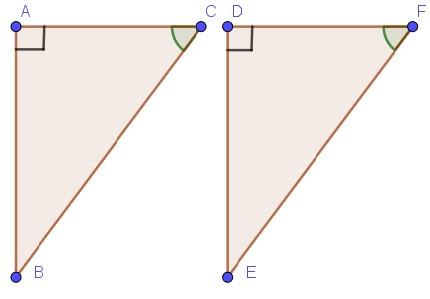Congruence and similarity between triangles can be connected, but they are not the same thing. Two or more triangles are similar if the correspondent sets of three angles are the same for the polygons. However, to be considered similar, the triangles do not need to have the same length for the sides.

The following image shows a pair of similar triangles. They have the same angles, but one has a smaller size compared to the other. If the triangle to the left was dilated, or the triangle to the right was shrunk till both reach the same size, they would be considered congruent.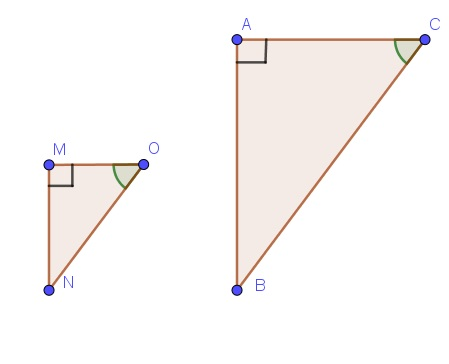Thus, congruent triangles are also similar triangles, but similar triangles are not always considered congruent.

Any transformations like rotation, translation, and reflection of any three-sided polygon from a set of congruent triangles will not affect their congruence.

Image 3 shows two congruent triangles translated with each other.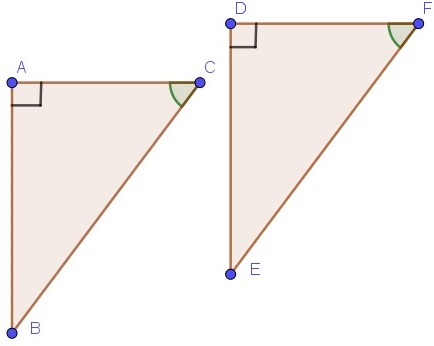Image 4 shows two congruent triangles where one is flipped upside down in relation to the other.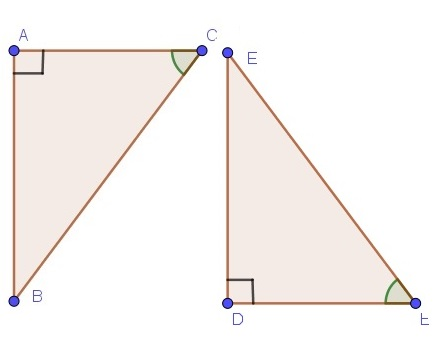Image 5 shows two congruent triangles with one flipped left to right (reflected) in relation to the other.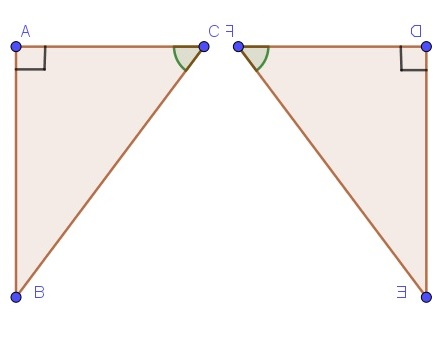Finally, image 6 shows two congruent triangles with one rotated 90 degrees in relation to the other.

## Stacking Triangles

This is Tricago.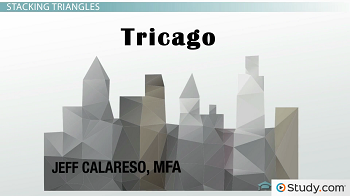Times are good in Tricago. Lots of new towers are being built. And each level in each tower is shaped like a triangle. That means that these triangles must by congruent. Congruent Triangles are triangles with three congruent sides and three congruent angles. If two of our triangle levels are congruent, we can always make them stack perfectly and neatly on top of each other.

But how do we know if they're congruent? Let's look at three statements that will tell us, based on what we know about the triangles' sides and angles.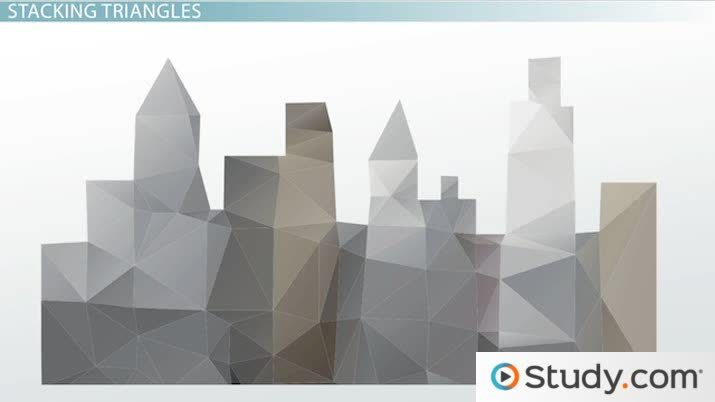An error occurred trying to load this video.

Try refreshing the page, or contact customer support.

Coming up next: Congruence Proofs: Corresponding Parts of Congruent Triangles

### You're on a roll. Keep up the good work!

Replay
Your next lesson will play in 10 seconds
• 0:05 Stacking Triangles
• 0:45 SSS Postulate
• 2:13 SAS Postulate
• 3:27 ASA Postulate
• 5:13 Lesson Summary
Save Save

Want to watch this again later?

Timeline
Autoplay
Autoplay
Speed Speed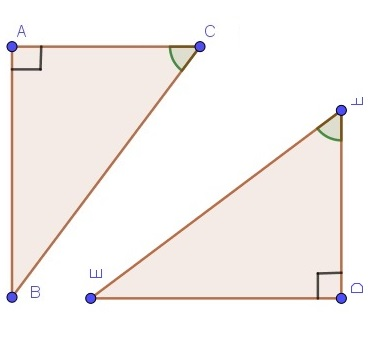## Triangle Congruence Postulates or Theorems

Knowing what determines the congruence between two triangles, one could simply go over all the angles and sides of the analyzed triangles. Then, they can determine if they are congruent or not.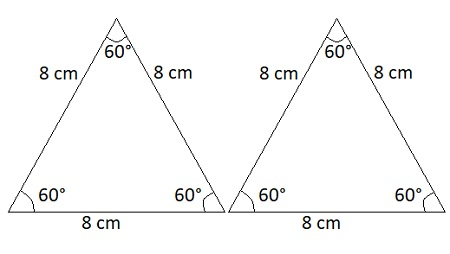The triangles shown in the previous image are congruent. They both have the same measures for sides and angles between each other. However, having the values of two out of the three angles for each triangle, one can calculate the other angle since the sum of the internal angles of a triangle is always 180 degrees. In some cases, not all the measurements for sides and angles will be given for each triangle. To solve this problem, geometry brings three postulates that are helpful to test the congruency between triangles. These are:

• ASA (angle-side-angle)
• SAS (side-angle-side)
• SSS (side-side-side)

## ASA Geometry or ASA Postulate

The angle-side-angle (ASA) postulate refers to the congruence between two pairs of correspondent angles and a pair of correspondent sides (between the angles) to prove the congruency between two triangles.

• Like the other postulates, the ASA can be used for more than two triangles. This lesson tests the congruency only between two of them for didactical purposes.

Example for postulate 1:

In the following image, the sides {eq}\overline {AC} {/eq} and {eq}\overline {DF} {/eq} are correspondent to each other, the angle {eq}\alpha {/eq} is correspondent to angle {eq}\theta {/eq}, and the angle {eq}\beta {/eq} is correspondent to the angle {eq}\phi {/eq}.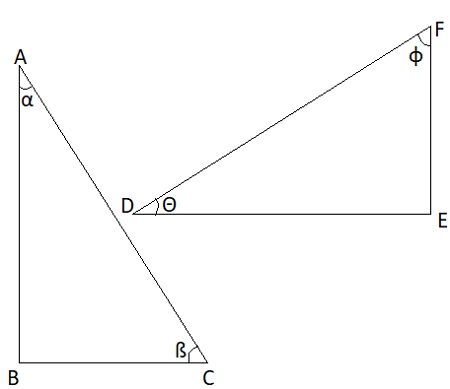The postulate ASA proves congruency between the two triangles if:

A: {eq}\alpha {/eq} = {eq}\theta {/eq}

S: {eq}\overline {AC} = \overline {DF} {/eq}

A: {eq}\beta {/eq} = {eq}\phi {/eq}

### ASA Triangle Congruence

Example with numbers:

Test the congruency between the triangles below using the postulate ASA.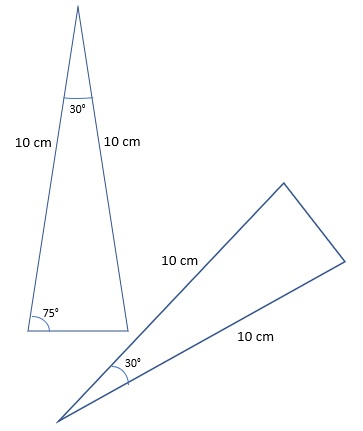The triangle to the left presents an ASA sequence of a 30 degrees angle, a 10 cm wide, and a 75 degrees angle. The triangle to the left presents a correspondent 30 degrees angle and a 10 cm side. Observing that the two remaining angles of this last triangle must have the same value, subtracting 30 degrees from 180, we have 150 degrees. Dividing it by two, the angles in the sequence will be 75 degrees. Thus, the two triangles are congruent.

## Side-Angle-Side Theorem: SAS Postulate

The side-angle-side (SAS) postulate refers to the congruency between two pairs of correspondent sides and a pair of correspondent angles (between those sides) to prove the congruency between triangles.

Example for postulate 2:

## SSS Postulate

First, there's the side-side-side postulate, or SSS. This states that if three sides of one triangle are congruent to three sides of another triangle, then the triangles are congruent. Should we see this in action? Okay, let's start with an equilateral triangle.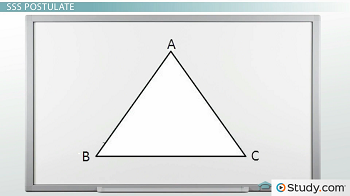But what if we add a line from A to the midpoint of BC? Let's call this AD. We now have two smaller triangles: ABD and ACD.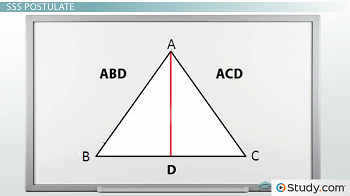Can we be sure? Well, since ABC is an equilateral triangle, we know angles B and C are each 60 degrees. And angles ADB and ADC are each 90 degrees. Then we split A into BAD and CAD, both of which are 30 degrees. So all three sides and all three angles match. So they are congruent!

So if we're trying to make sure the levels of our building will match, we can measure the three sides. Without even looking at the angles, if the sides all match, the SSS postulate tells us they must be congruent.

## SAS Postulate

The SSS postulate is great, but measuring all three sides involves a lot of walking around big triangles. What if you're tired? Is there a shorter way? Yes! There's the Side-Angle -Side postulate, or SAS. This states that if two sides and the included angle of one triangle are congruent to two sides and the included angle of another triangle, then the triangles are congruent.

Here are two triangles.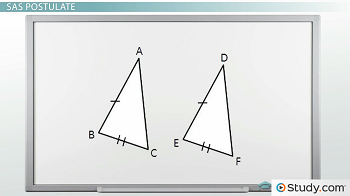That's two sides and the included angle. Using the SAS postulate, we know they're congruent. Note that the postulate stipulates the included angle. That means the angle formed by the two sides. If we only knew that angles A and D were equal, that wouldn't be enough. Fortunately, we stopped to measure angles B and E while we were walking around, so we're good.

## ASA Postulate

Wait, Tricago is a city of threes, and that's only two postulates. Can we get a third one? It just wouldn't be Tricago if they didn't come in threes.

To unlock this lesson you must be a Study.com Member.

Video Transcript

## Stacking Triangles

This is Tricago.Times are good in Tricago. Lots of new towers are being built. And each level in each tower is shaped like a triangle. That means that these triangles must by congruent. Congruent Triangles are triangles with three congruent sides and three congruent angles. If two of our triangle levels are congruent, we can always make them stack perfectly and neatly on top of each other.

But how do we know if they're congruent? Let's look at three statements that will tell us, based on what we know about the triangles' sides and angles.

## SSS Postulate

First, there's the side-side-side postulate, or SSS. This states that if three sides of one triangle are congruent to three sides of another triangle, then the triangles are congruent. Should we see this in action? Okay, let's start with an equilateral triangle.But what if we add a line from A to the midpoint of BC? Let's call this AD. We now have two smaller triangles: ABD and ACD.Can we be sure? Well, since ABC is an equilateral triangle, we know angles B and C are each 60 degrees. And angles ADB and ADC are each 90 degrees. Then we split A into BAD and CAD, both of which are 30 degrees. So all three sides and all three angles match. So they are congruent!

So if we're trying to make sure the levels of our building will match, we can measure the three sides. Without even looking at the angles, if the sides all match, the SSS postulate tells us they must be congruent.

## SAS Postulate

The SSS postulate is great, but measuring all three sides involves a lot of walking around big triangles. What if you're tired? Is there a shorter way? Yes! There's the Side-Angle -Side postulate, or SAS. This states that if two sides and the included angle of one triangle are congruent to two sides and the included angle of another triangle, then the triangles are congruent.

Here are two triangles.That's two sides and the included angle. Using the SAS postulate, we know they're congruent. Note that the postulate stipulates the included angle. That means the angle formed by the two sides. If we only knew that angles A and D were equal, that wouldn't be enough. Fortunately, we stopped to measure angles B and E while we were walking around, so we're good.

## ASA Postulate

Wait, Tricago is a city of threes, and that's only two postulates. Can we get a third one? It just wouldn't be Tricago if they didn't come in threes.

To unlock this lesson you must be a Study.com Member.

#### What is SSS, AAS, SAS, and ASA?

They are postulates to test if two triangles are congruent.

1. SSS refers to the equality of three sides between triangles.
2. AAS refers to the equality between two sides and an angle between triangles.
3. SAS refers to the equality between two sides and an angle (between the sides) between triangles.
4. ASA refers to the equality between two angles and one side between triangles.

#### How to know if it's ASA or AAS?

The position of the side is different for ASA and AAS postulates. In the former, the side connects the two angles in question. In the latter, the side comes after the sequence of angles.

#### Is ASA a real geometry postulate?

ASA is a geometry postulate that tests if two triangles are congruent. According to this postulate, if one triangle has two angles and a side (that connects the angles) equal to the correspondent ones of another triangle, then these two triangles will be congruent.

#### What is the difference between SAS and SSS postulate?

SAS refers to the congruency between two pairs of sides and one pair of angles. Whereas, SSS refers to the congruency between three pairs of sides.

### Register to view this lesson

Are you a student or a teacher?

Back

### Resources created by teachers for teachers

Over 30,000 video lessons & teaching resources‐all in one place.Video lessonsQuizzes & WorksheetsClassroom IntegrationLesson Plans

I would definitely recommend Study.com to my colleagues. It’s like a teacher waved a magic wand and did the work for me. I feel like it’s a lifeline.

Jennifer B.
TeacherCreate an account to start this course today
Used by over 30 million students worldwide